# RS Aggarwal Solutions for Class 6 Maths Chapter 23 Pictograph

RS Aggarwal Solutions for Class 6 Chapter 23 Pictograph are provided here through simple step by step explanations. RS Aggarwal textbook solutions will help the students to revise the complete syllabus and score more marks in examinations. Students who aim to understand chapter 23 effectively are advised to practice RS Aggarwal textbook solutions thoroughly.

BYJU’S experts have solved RS Aggarwal textbook solutions in detail so that students can grasp them easily. Download PDF of Class 6 Chapter 23 in their respective links.

## Download PDF of RS Aggarwal Solutions for Class 6 Maths Chapter 23 Pictograph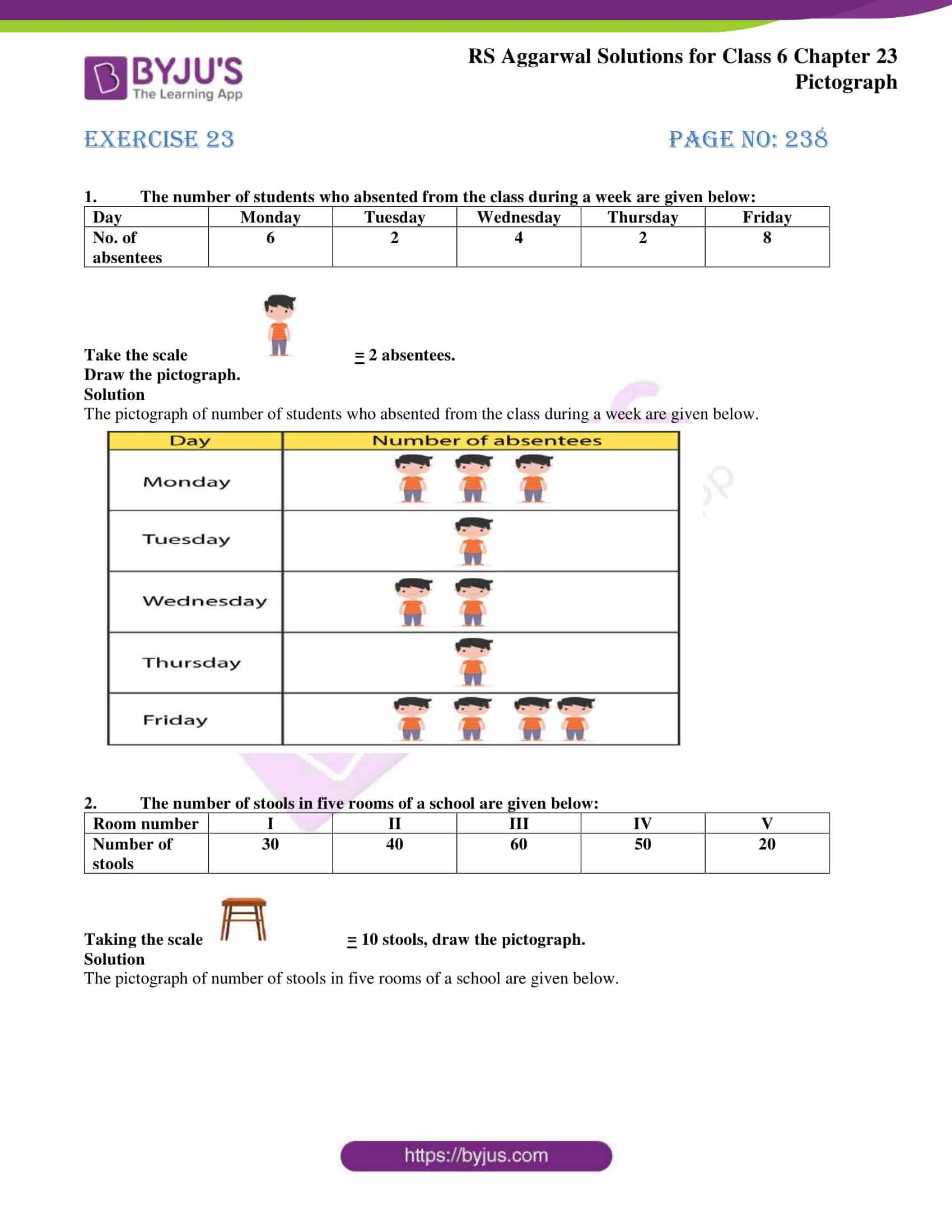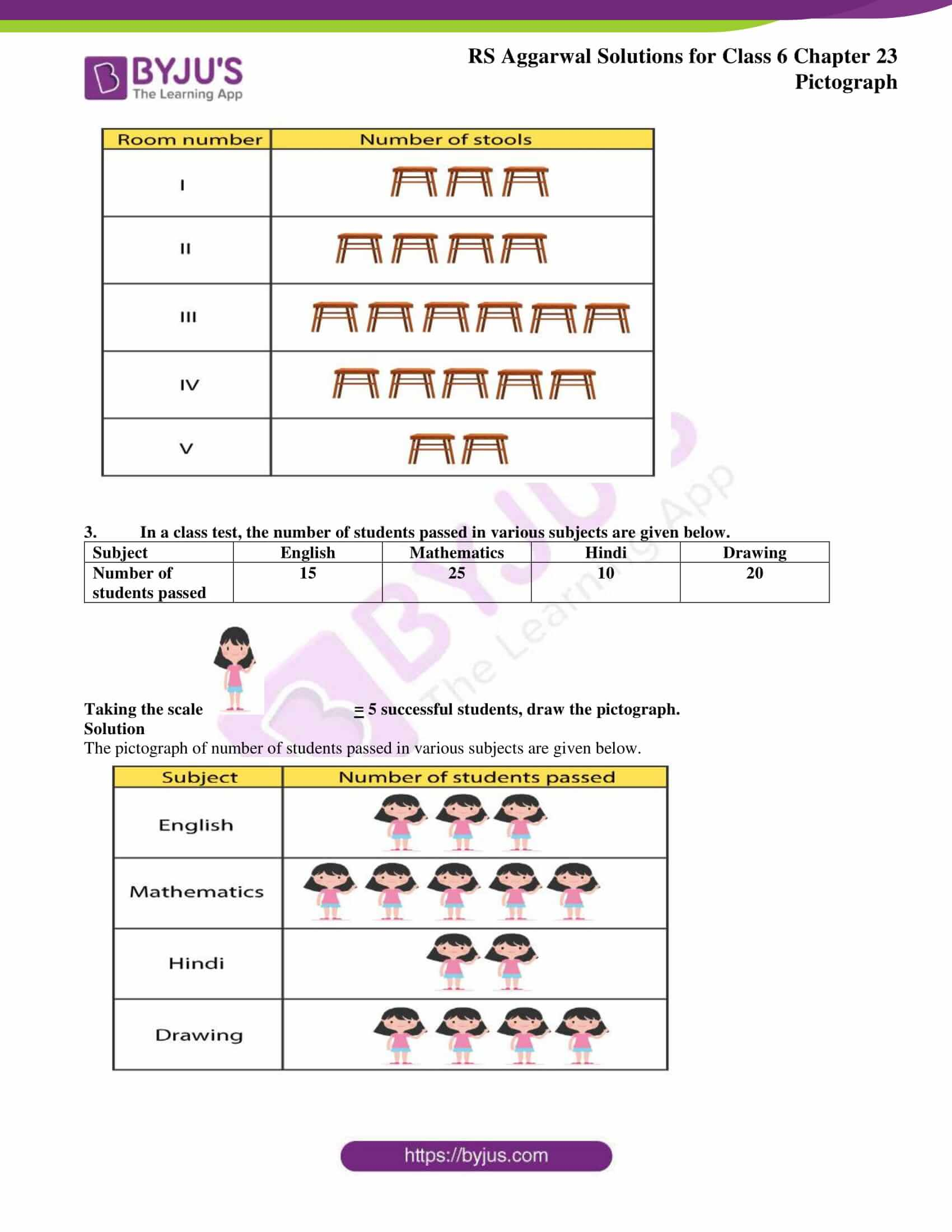## Exercise 23 PAGE NO: 238

1. The number of students who absented from the class during a week are given below:

 Day Monday Tuesday Wednesday Thursday Friday No. of absentees 6 2 4 2 8

Take the scale= 2 absentees.

Draw the pictograph.

Solution

The pictograph of number of students who absented from the class during a week are given below.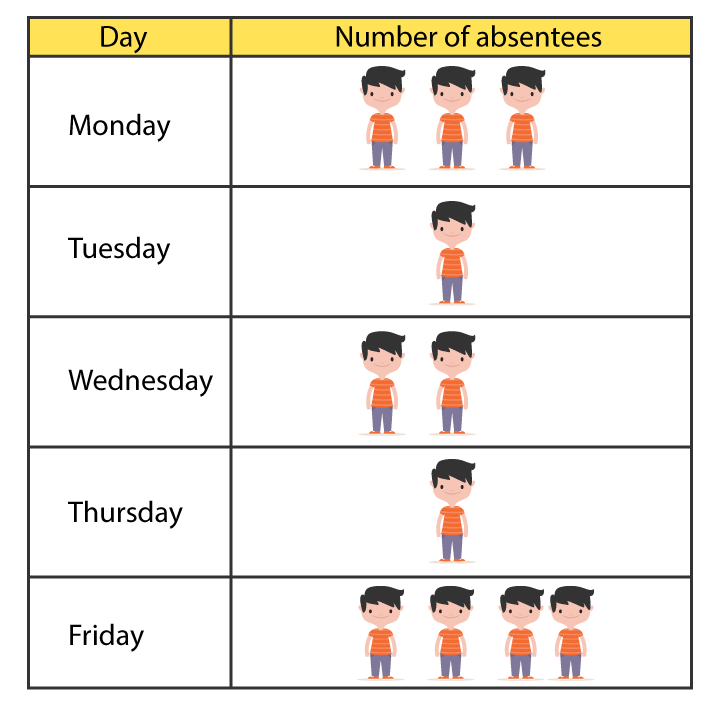2. The number of stools in five rooms of a school are given below:

 Room number I II III IV V Number of stools 30 40 60 50 20

Taking the scale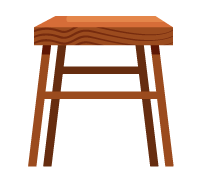= 10 stools, draw the pictograph.

Solution

The pictograph of number of stools in five rooms of a school are given below.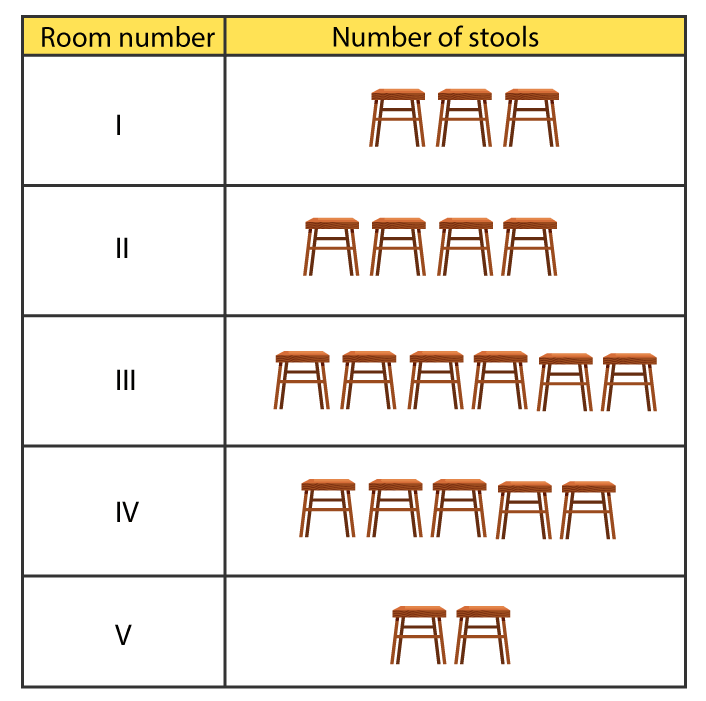3. In a class test, the number of students passed in various subjects are given below.

 Subject English Mathematics Hindi Drawing Number of students passed 15 25 10 20

Taking the scale= 5 successful students, draw the pictograph.

Solution

The pictograph of number of students passed in various subjects are given below.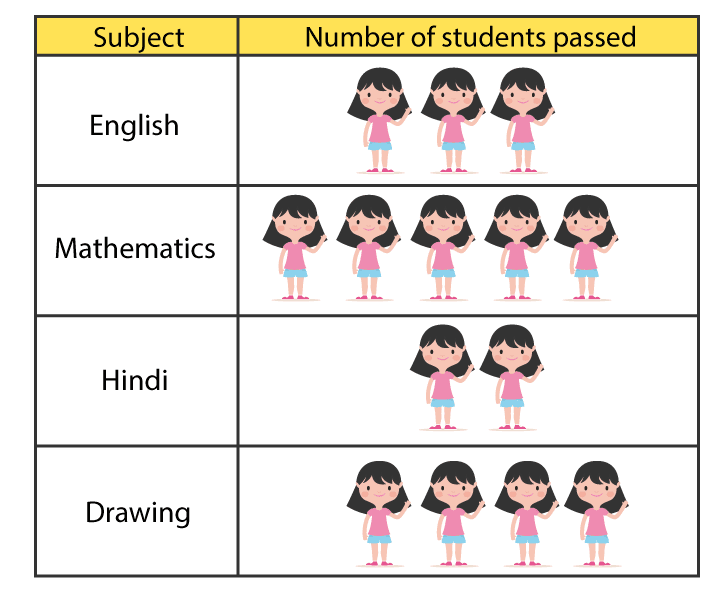### RS Aggarwal Solutions for Class 6 Chapter 23 Pictograph

Chapter 23 has 1 exercise. Solutions have been solved in depth for all questions from this chapter of RS Aggrawal textbook for students who want to ace the examination. Let’s have a look at the topic mentioned in this chapter.

• Pictograph

Numerical information in graph by using pictures, symbols to represent data sets is known as Pictograph. The cigarette with a red circle and slash around it which shows no smoking is an example of Pictograph.